# PSEB 7th Class Maths Solutions Chapter 10 Practical Geometry Ex 10.3

Punjab State Board PSEB 7th Class Maths Book Solutions Chapter 10 Practical Geometry Ex 10.3 Textbook Exercise Questions and Answers.

## PSEB Solutions for Class 7 Maths Chapter 10 Practical Geometry Ex 10.3

1. Construct ΔABC such that AB = 4 cm, ∠B = 30°, BC = 4 cm. Also name the type of triangle on the basis of sides.
Solution:
Given : Two sides of ΔABC as AB = 4 cm, BC = 4 cm and ∠B = 30°.
To construct: A triangle with these two sides and included angle.
Step of Construction :
Step 1. We first draw a rough sketch of the ΔABC and indicate the measure of these two sides and included angle.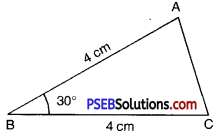Step 2. Draw a line segment BC of length 4 cm.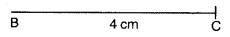Step 3. At B draw BX making an angle of 30° with BC (The point A must be somewhere on this ray of the angle).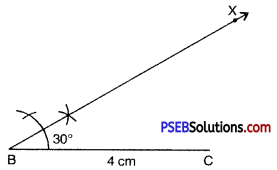Step 4. (To fix A, the distance AB has been given) With B as centre, draw an arc of radius 3 cm. It cuts BX at the point A.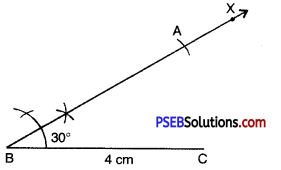Step 5. Join AC.
ΔDEF is now obtained.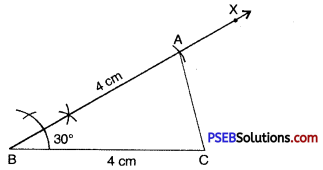Since two sides of triangle are equal.
Therefore ΔABC is an isosceles triangle.2. Construct ΔABC with AB = 7.5 cm, BC = 5 cm and ∠B = 30°.
Solution:
Given. Two sides of ΔABC as AB = 7.5 cm,
BC = 5 cm
and ∠B = 30°
To construct A triangle with these two sides and included angle.
Steps of Construction.
Step 1. We first draw a rough sketch of the ΔABC and indicate the measures of these two sides and included angle.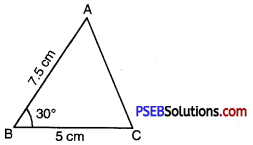Step 2. Draw a line segment BC of length 5 cm.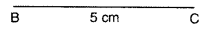Step 3. At B draw BX making an angle of 30° with BC. (The point A must be somewhere on this ray of the angle)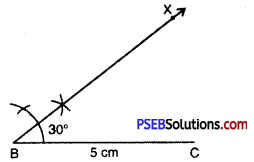Step 4. (To fix A; the distance BC has been given) With B as centre draw an arc of radius 7.5 cm. It cuts CX at the point A.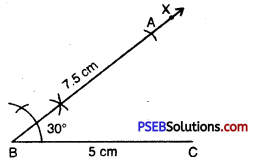Step 5 : Join AC.
ΔABC is now obtained.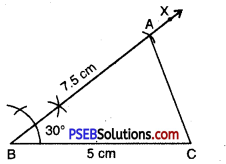3. Construct a triangle XYZ, such that XY = 6 cm, YZ = 6 cm and ∠Y = 60°. Also name the type of this triangle.
Solution:
Step 1. Draw a rough sketch of XYZ with given measures.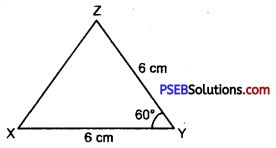Step 2. Draw a line segment XY of length 6 cm.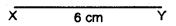Step 3. With the help of compass, at Y, draw a ray YA making an angle 60°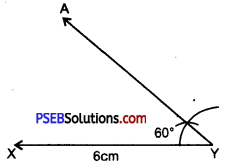Step 4. With Y as centre and radius 6 cm. draw an arc intersecting the ray YX at point Z.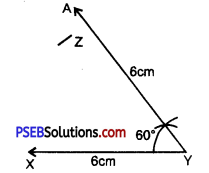Step 5. Join XZ.ΔXYZ is required triangle, Measure the third side. We see that ZX = 6 cm
∴ In Δ XYZ
XY = YZ = ZX = 6 cm
Therefore XYZ is an equilateral triangle.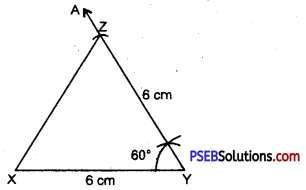4. Which of the following triangle can be constructed using SAS criterion.
(a) AB = 5 cm, BC = 5 cm, CA = 6 cm
(b) AB = 5 cm, BC = -5 cm, ∠B = 40°
(c) ∠A = 60°, ∠B = 60°, ∠C = 60°
(d) BC = 5 cm, ∠B = ∠C = 45°